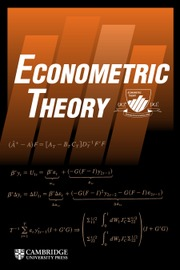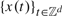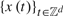Skip to main content Accessibility help
Home
Hostname: page-component-77ffc5d9c7-wmdqp Total loading time: 0.259 Render date: 2021-04-23T17:06:36.447Z Has data issue: true Feature Flags: { "shouldUseShareProductTool": true, "shouldUseHypothesis": true, "isUnsiloEnabled": true, "metricsAbstractViews": false, "figures": false, "newCiteModal": false, "newCitedByModal": true }Econometric Theory

# SPECIFICATION TESTS FOR LATTICE PROCESSES

Published online by Cambridge University Press:  19 August 2014

Corresponding
E-mail address:

## Abstract

We consider an omnibus test for the correct specification of the dynamics of a sequence$\left\{ {x\left( t \right)} \right\}_{t \in Z^d }$ in a lattice. As it happens with causal models and d = 1, its asymptotic distribution is not pivotal and depends on the estimator of the unknown parameters of the model under the null hypothesis. One first main goal of the paper is to provide a transformation to obtain an asymptotic distribution that is free of nuisance parameters. Secondly, we propose a bootstrap analog of the transformation and show its validity. Thirdly, we discuss the results when$\left\{ {x\left( t \right)} \right\}_{t \in Z^d }$ are the errors of a parametric regression model. As a by product, we also discuss the asymptotic normality of the least squares estimator of the parameters of the regression model under very mild conditions. Finally, we present a small Monte Carlo experiment to shed some light on the finite sample behavior of our test.

Type
ARTICLES
Information
Econometric Theory , April 2015 , pp. 294 - 336
Copyright
Copyright © Cambridge University Press 2014

## Access options

Get access to the full version of this content by using one of the access options below.

## References

Baltagi, B., Kelejian, H.H., & Prucha, I.R. (eds.) (2007) Analysis of spatially dependent data. Annals issue. Journal of Econometrics, Annals Issue, 140.Google Scholar
Batchelor, L.I.A. & Reed, H.S. (1924) Relation of the variability of yields of fruit trees to the accuracy of field trials. Journal of Agricultural Research 12, 245283.Google Scholar
Besag, J. (1974) Spatial interaction and the statistical analysis of lattice systems (with discussion). Journal of the Royal Statistical Society, Series B 36, 192236.Google Scholar
Bickel, P.J. & Wichura, M.J. (1971) Convergence criteria for multiparameter stochastic processes and its applications. Annals of Mathematical Statistics 42, 16561670.CrossRefGoogle Scholar
Billingsley, P. (1968) Convergence of Probability Measures. Wiley.Google Scholar
Bloomfield, P. (1973) An exponential model for the spectrum of a scalar time series. Biometrika 61, 217226.CrossRefGoogle Scholar
Bolthausen, E. (1982) On the central limit theorem for stationary mixing random fields. The Annals of Probability 10, 10471050.CrossRefGoogle Scholar
Brillinger, D.R. (1981) Time Series, Data Analysis and Theory. Holden-Day.Google Scholar
Brown, R.L., Durbin, J., & Evans, J.M. (1975) Components of Cramér-von Mises statistics II. Journal of the Royal Statistical Society, Series B 37, 216237.Google Scholar
Chen, H. & Romano, J.P. (1999) Bootstrap-assisted goodness-of-fit tests in the frequency domain. Journal of Time Series Analysis 20, 619654.CrossRefGoogle Scholar
Cressie, N.A.C. (1993) Statistics for Spatial Data. Wiley.Google Scholar
Cressie, N. & Huang, H.C. (1999) Classes of nonseparable, spatio-temporal stationary covariance functions. Journal of the American Statistical Association 94, 13301340.CrossRefGoogle Scholar
Crujeiras, R.M., Fernandez-Casal, R., & Gonzalez-manteiga, W. (2008) An L 2-test for comparing spatial spectral densities. Statistics & Probability Letters 78, 25432551.CrossRefGoogle Scholar
Dahlhaus, R. & Künsch, H. (1986) Edge effect and efficient parameter estimation for stationary random fields. Biometrika 74, 877882.CrossRefGoogle Scholar
Davis, R.A., Alexandre Trindade, A., & Breidt, F.J. (2001) Least absolute deviation estimation for all-pass time series models. Annals of Statistics 29, 919946.Google Scholar
Delgado, M.A., Hidalgo, J., & Velasco, C. (2005) Distribution free goodness-of-fit tests for linear processes. Annals of Statistics 33, 25682609.CrossRefGoogle Scholar
Delgado, M.A., Hidalgo, J., & Velasco, C. (2011) Bootstrap assisted specification tests for the Farima model. Econometric Theory 27, 10831116.CrossRefGoogle Scholar
Eubank, R.L. & LaRicca, V. (1992) Asymptotic comparison of Cramér-von Mises and nonparametric techniques for testing goodness-of-fit. Annals of Statistics 20, 20712086.CrossRefGoogle Scholar
Fernandez-Casal, R., Gonzalez-Manteiga, W., & Febrero-Bande, M. (2003) Flexible spatio-temporal stationary variogram models. Statistics and Computing 13, 127136.CrossRefGoogle Scholar
Genton, M.G. & Koul, H.L. (2008) Minimum distance inference in unilateral autoregressive lattice processes. Statistica Sinica 18, 617631.Google Scholar
Giacomini, R., Politis, D.N., & White, H. (2013) A warp-speed method for conducting Monte Carlo experiments involving bootstrap estimators. Econometric Theory 29, 567609.CrossRefGoogle Scholar
Grenander, U. (1950) Stochastic processes and statistical inference. Arkiv för Matematik 1, 195277.CrossRefGoogle Scholar
Guyon, X. (1982) Parameter estimation for a stationary process on a 𝑑-dimension lattice. Biometrika 69, 95105.CrossRefGoogle Scholar
Hainz, G. & Dahlhaus, R. (2000) Spectral domain bootstrap tests for stationary time series. Preprint.
Hannan, E.J. (1970) Multiple Time Series. Wiley.CrossRefGoogle Scholar
Hannan, E.J. (1973) The asymptotic theory of linear time series models. Journal of Applied Probability 10, 130145.CrossRefGoogle Scholar
Hidalgo, J. (2009) Goodness of fit for lattice processes. Journal of Econometrics 151, 113128.CrossRefGoogle Scholar
Hidalgo, J. & Kreiss, J.P. (2006) Bootstrap specification tests for linear covariance stationary processes. Journal of Econometrics 133, 807839.CrossRefGoogle Scholar
Hong, Y. (1996) Consistent testing for serial-correlation of unknown form. Econometrica 64, 837864.CrossRefGoogle Scholar
Ibragimov, I.A. & Rozanov, Y.A. (1978) Gaussian Random Processes. Springer-Verlag.CrossRefGoogle Scholar
Jenish, N. & Prucha, I.R. (2009) Central limit theorems and uniform laws of large numbers for arrays of random fields. Journal of Econometrics 150, 8698.CrossRefGoogle ScholarPubMed
Khmaladze, E.V. (1981) Martingale approach in the theory of goodness-of-fit tests. Theory of Probability and Applications 26, 240257.CrossRefGoogle Scholar
Lanne, M. & Saikonnen, P. (2011) Noncausal autoregressive for economic time series. Journal of Time Series Econometrics 3, 130.CrossRefGoogle Scholar
Lanne, M. & Saikonnen, P. (2013) Noncausal vector autoregression. Econometric Theory 29, 447481.CrossRefGoogle Scholar
Mardia, K.V. & Marshall, R.J. (1984) Maximum likelihood estimation of models for residual covariance in spatial regression. Biometrika 71, 135146.CrossRefGoogle Scholar
McKeague, I.W., Nikabadze, A.M., & Sun, Y. (1995) An omnibus test for independence of survival time from a covariate. Annals of Statistics 223, 450475.CrossRefGoogle Scholar
Mercer, W.B. & Hall, A.D. (1911) The experimental error of field trials. Journal of Agricultural Science 4, 107132.CrossRefGoogle Scholar
Mitchell, M.W., Genton, M.G., & Gumpertz, M.L. (2005) Testing for separability of space–time covariances. Environmetrics 16, 819831.CrossRefGoogle Scholar
Neyman, J. (1937) Smooth test for goodness of fit. Arkiv for matematik 20, 150199.Google Scholar
Paparoditis, E. (2000) Spectral density based goodness-of-fit tests for time series models. Scandinavian Journal of Statistics 27, 143176.CrossRefGoogle Scholar
Robinson, P.M. (1988) The stochastic difference between econometric statistics. Econometrica 56, 531548.CrossRefGoogle Scholar
Robinson, P.M. (2012) Inference on power law spatial trends. Bernoulli 18, 644677.CrossRefGoogle Scholar
Robinson, P.M. & Thawornkaiwong, S. (2012) Statistical inference on regressing with spatial dependence. Journal of Econometrics 167, 521542.CrossRefGoogle Scholar
Robinson, P.M. & Vidal-Sanz, J. (2006) Modified Whittle estimation of multilateral models on a lattice. Journal of Multivariate Analysis 60, 234349.Google Scholar
Schoenfeld, D.A. (1977) Asymptotic properties of tests based on linear combinations of the Cramér-von Mises statistic. Annals of Statistics 5, 10171026.CrossRefGoogle Scholar
Shao, J. & Tu, D. (1995) The Jackknife and Bootstrap. Springer-Verlag.CrossRefGoogle Scholar
Solo, V. (1986) Modeling of two-dimensional random field by parametric Cepstrum. IEEE Transactions on Information Theory 32, 743750.CrossRefGoogle Scholar
Stute, W. (1997) Nonparametric model checks for regression. Annals of Statistics 25, 613641.Google Scholar
Whittle, P. (1954) On stationary processes in the plane. Biometrika 41, 434449.CrossRefGoogle Scholar
Yajima, Y. & Matsuda, Y. (2011) Asymptotic properties of the LSE of a spatial regression in both weakly and strongly dependent stationary random fields. Preprint. University of Tokyo.
Zhu, J., Huang, H.C., & Reyes, P.E. (2010) On selection of spatial linear models for lattice data. Journal of the Royal Statistical Society, Series B 72, 389402.CrossRefGoogle Scholar

### Full text views

Full text views reflects PDF downloads, PDFs sent to Google Drive, Dropbox and Kindle and HTML full text views.

Total number of HTML views: 0
Total number of PDF views: 100 *
View data table for this chart

* Views captured on Cambridge Core between September 2016 - 23rd April 2021. This data will be updated every 24 hours.

# Send article to Kindle

To send this article to your Kindle, first ensure no-reply@cambridge.org is added to your Approved Personal Document E-mail List under your Personal Document Settings on the Manage Your Content and Devices page of your Amazon account. Then enter the ‘name’ part of your Kindle email address below. Find out more about sending to your Kindle. Find out more about sending to your Kindle.

Note you can select to send to either the @free.kindle.com or @kindle.com variations. ‘@free.kindle.com’ emails are free but can only be sent to your device when it is connected to wi-fi. ‘@kindle.com’ emails can be delivered even when you are not connected to wi-fi, but note that service fees apply.

Find out more about the Kindle Personal Document Service.

SPECIFICATION TESTS FOR LATTICE PROCESSES
Available formats
×

# Send article to Dropbox

To send this article to your Dropbox account, please select one or more formats and confirm that you agree to abide by our usage policies. If this is the first time you use this feature, you will be asked to authorise Cambridge Core to connect with your <service> account. Find out more about sending content to Dropbox.

SPECIFICATION TESTS FOR LATTICE PROCESSES
Available formats
×

# Send article to Google Drive

To send this article to your Google Drive account, please select one or more formats and confirm that you agree to abide by our usage policies. If this is the first time you use this feature, you will be asked to authorise Cambridge Core to connect with your <service> account. Find out more about sending content to Google Drive.

SPECIFICATION TESTS FOR LATTICE PROCESSES
Available formats
×
×

#### Conflicting interests

Do you have any conflicting interests? *